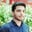Trusted answers to developer questions
Trusted Answers to Developer Questions

Related Tags

r
communitycreator

# What is the median() method in R?Salman Yousaf

Grokking Modern System Design Interview for Engineers & Managers

Ace your System Design Interview and take your career to the next level. Learn to handle the design of applications like Netflix, Quora, Facebook, Uber, and many more in a 45-min interview. Learn the RESHADED framework for architecting web-scale applications by determining requirements, constraints, and assumptions before diving into a step-by-step design process.

In R, the median() method is used to find the median of the sample data, with the assumption that the dataset or sample data is sorted from smallest to largest.

1. Odd length: if the number of entries in the dataset is odd, then the middle value will be the median.
2. Even length: if the number of entries in the dataset is even, then the median will be the average of two middle values.

### Syntax

The syntax for the median() function to calculate the middle value of the vector x is as follows:

median(x, na.rm = FALSE,...)


### Parameters

• x: vector of numerical values.
• na.rm: default=False. na.rm is a boolean field that can either be True or False. If it is set to False, the dataset does not contain NA values. Otherwise, we can set it to True.
• ...: further potential arguments for methods.

### Return value

• Object: The default method will return a one-length object of the same type as x.
• Double integer: If x is an even-length integer, the return type will be double.
• NA: If a dataset contains NA values and na.rm is set to False.

### Code

The code snippet below illustrates how the median() method works with a single argument. It takes x to compute the median of these values.

# Create the vector.
x <- c(1,2,3,4,5.3,6,7,8,9,13,11)

# Find the median.
mid_value <- median(x)
cat("Median is:", mid_value)

In the example below, the median() method takes a vector x and na.rm set to TRUE. median() will first eliminate NA values and then it will compute the median of the remaining values.

# Create the vector.
x <- c(1,2,3,4,5.3,6,7,8,9,NA,13,11)

# Find the median.
mid_value <- median(x,na.rm=TRUE)
print(mid_value)

RELATED TAGS

r
communitycreator

CONTRIBUTORSalman Yousaf

Grokking Modern System Design Interview for Engineers & Managers

Ace your System Design Interview and take your career to the next level. Learn to handle the design of applications like Netflix, Quora, Facebook, Uber, and many more in a 45-min interview. Learn the RESHADED framework for architecting web-scale applications by determining requirements, constraints, and assumptions before diving into a step-by-step design process.

Keep Exploring

Learn in-demand tech skills in half the time